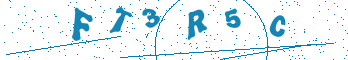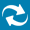# Xilinx System Generator with Active-HDL

The Active-HDL System Generator interface provides a way to incorporate custom HDL models into the Xilinx System Generator. This enables user to co-simulate Xilinx System Generator blocks in Mathwork's Simulink with custom HDL blocks in Active-HDL.

Please read the requirements and limitation from Xilinx System Generator/Black Box help and pay special attention to the rules on entity/module coding, clocking and data types

## Preparing VHDL model in Active-HDL.

We will create a ‘clocked_multiplier” design using IPCore Wizard of Active-HDL.

The figure below shows the BDE schematic file clocked_multiplier.bde created in Active-HDL.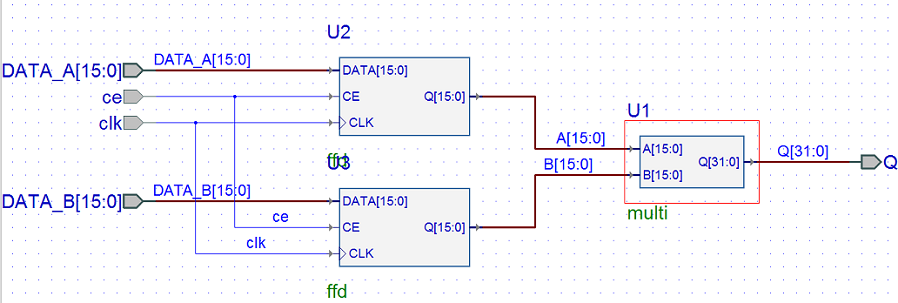ffd VHDL code:

```library IEEE;
use IEEE.std_logic_1164.all;
entity ffd is
port (
CE : in std_logic;
CLK : in std_logic;
DATA : in std_logic_vector(15 downto 0);
Q : out std_logic_vector(15 downto 0)
);
end entity;
--}} End of automatically maintained section
architecture ffd_arch of ffd is
begin
process (CLK)
begin
if rising_edge(CLK) then
if CE = '1' then
Q <= DATA;
end if;
end if;
end process;
end ffd_arch;
```

multi VHDL code:

```library IEEE;
use IEEE.std_logic_1164.all;
entity multi is
port
(	A : in std_logic_vector(15 downto 0);
B : in std_logic_vector(15 downto 0);
Q : out std_logic_vector(31 downto 0)
);
end multi;
--}} End of automatically maintained section
library IEEE;
use IEEE.std_logic_signed.all;
architecture multi_arch of multi is
begin
Q <= A * B;
end multi_arch;
```

clocked_multiplier VHDL code:

```library IEEE;
use IEEE.std_logic_1164.all;
entity clocked_multiplier is
port(
ce : in STD_LOGIC;
clk : in STD_LOGIC;
DATA_A : in STD_LOGIC_VECTOR(15 downto 0);
DATA_B : in STD_LOGIC_VECTOR(15 downto 0);
Q : out STD_LOGIC_VECTOR(31 downto 0)
);
end clocked_multiplier;
architecture clocked_multiplier of clocked_multiplier is
---- Component declarations -----
component ffd
port (
CE : in STD_LOGIC;
CLK : in STD_LOGIC;
DATA : in STD_LOGIC_VECTOR(15 downto 0);
Q : out STD_LOGIC_VECTOR(15 downto 0)
);
end component;
component multi
port (
A : in STD_LOGIC_VECTOR(15 downto 0);
B : in STD_LOGIC_VECTOR(15 downto 0);
Q : out STD_LOGIC_VECTOR(31 downto 0)
);
end component;

---- Signal declarations used on the diagram ----
signal A : STD_LOGIC_VECTOR (15 downto 0);
signal B : STD_LOGIC_VECTOR (15 downto 0);

begin
----  Component instantiations  ----
U1 : multi
port map(
A => A,
B => B,
Q => Q
);
U2 : ffd
port map(
CE => CE,
CLK => CLK,
DATA => DATA_A,
Q => A
);
U3 : ffd
port map(
CE => CE,
CLK => CLK,
DATA => DATA_B,
Q => B
);
end clocked_multiplier;
```

## Preparing to do the Co-simulation in Active-HDL

To start the co-simulation process in MATLAB’s Simulink environment, first you need to generate the Simulink description file (.m) for the top level entity or module of your HDL model . Active-HDL can do this automatically. To do that follow these steps in Active-HDL:

1. Open a workspace and set an active design that contains HDL unit(s) to be co-simulated.

2. Compile your HDL files representing the model you are going to co-simulate in Simulink (in our case it is clocked_multiplier.vhd)

3. Expand a source file (e.g. clocked_multiplier.vhd) that contains the top level design unit that will be used as a HDL black-box in Simulink. Right-click on this unit (e.g. clocked_multiplier(clocked_multiplier)) and select the Generate Block Description for Simulink option from the pop-up menu.

This option is unavailable if the design library is empty.4. Press Save to complete the MATLAB M-File generation.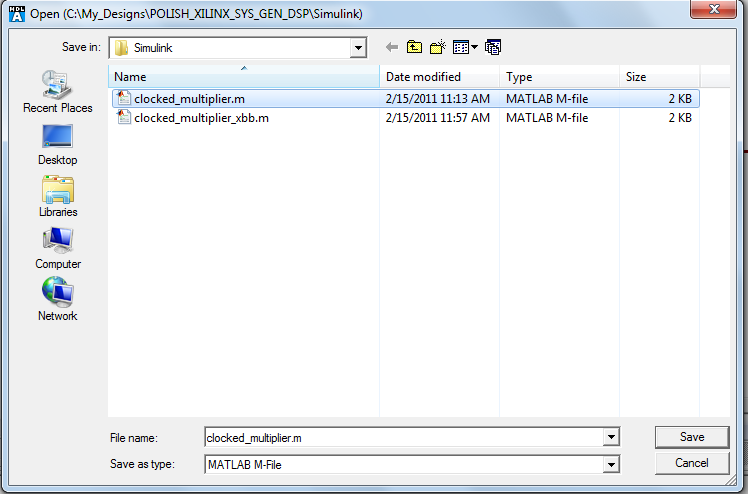You will see the following messages in the console:

# Generating block description file: 'C:\My_Designs\POLISH_XILINX_SYS_GEN_DSP\Simulink\clocked_multiplier.m'...

# Generation of block description file for "clocked_multiplier (clocked_multiplier)" completed successfully.

## Pure System Generator design

1. Open a new MATLAB SIMULINK model and begin adding the items presented in the following figures. Start with the signal generators and use the parameters shown.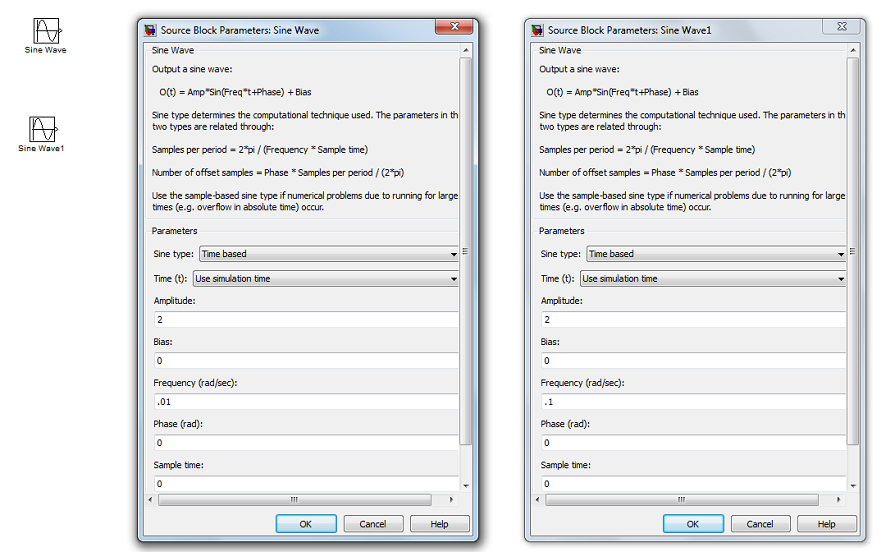2. Add the Gateway(s) and a scope and connect as shown.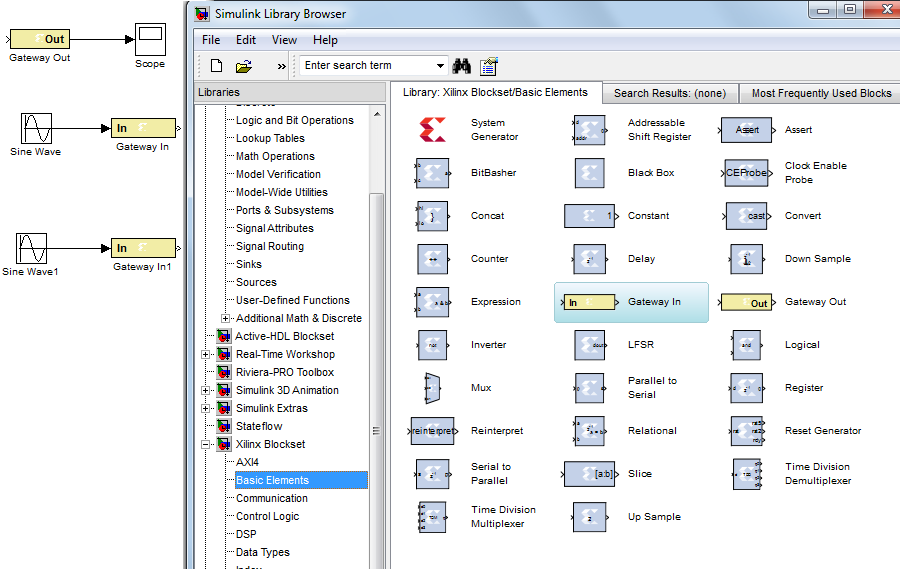3. Add the Xilinx System Generator and use the parameters shown.4. Add the Xilinx Multiplier Block and use the parameters shown, duplicate all connections shown in the figure.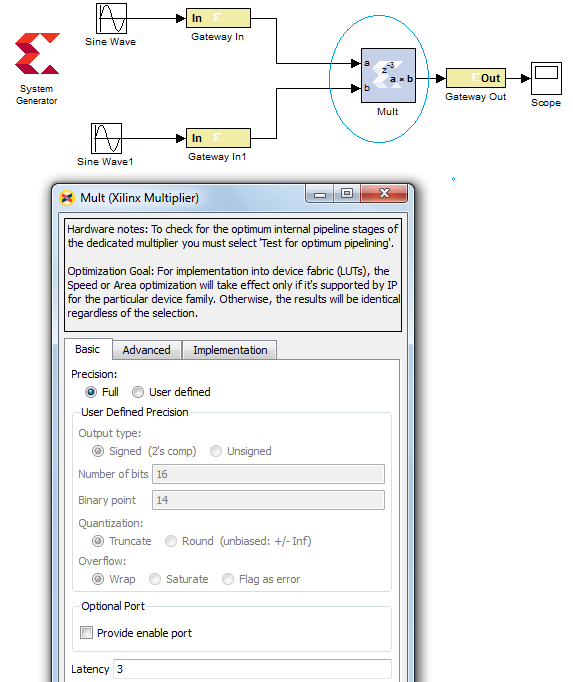5. Add the Active-HDL Co-simulation Block and use the parameters shown.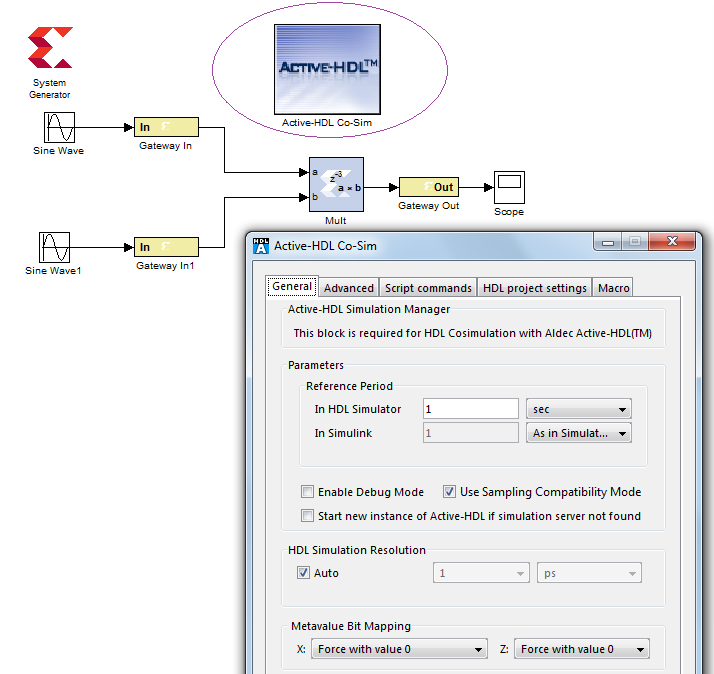6. Add the Active-HDL System Generation Black Box and select the ".m" file generated earlier. Click OK.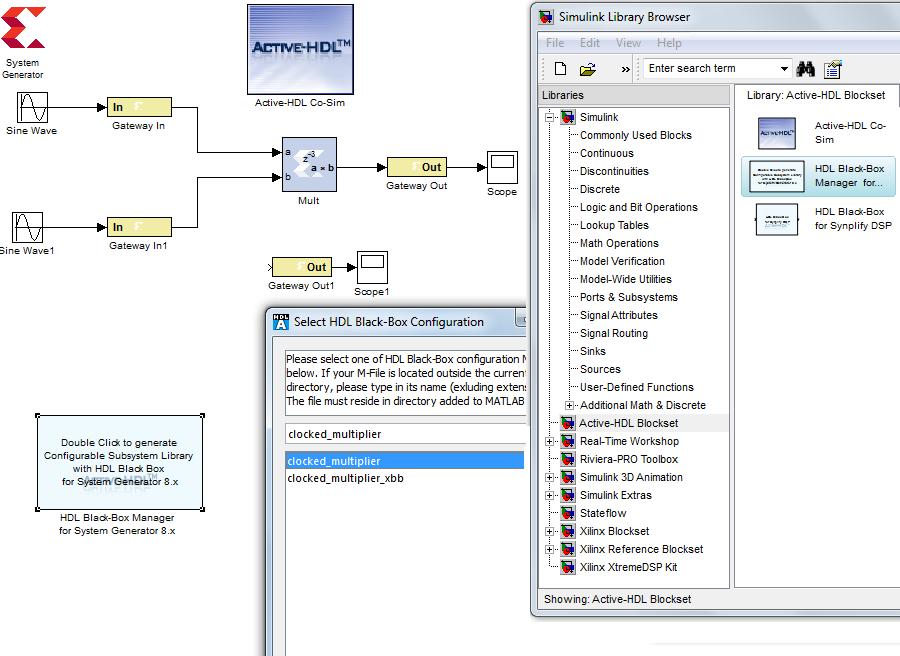7. Click on the Active-HDL System Generation Black Box to open the box if it does not open automatically. Select the Input Ports tab and make the changes indicated.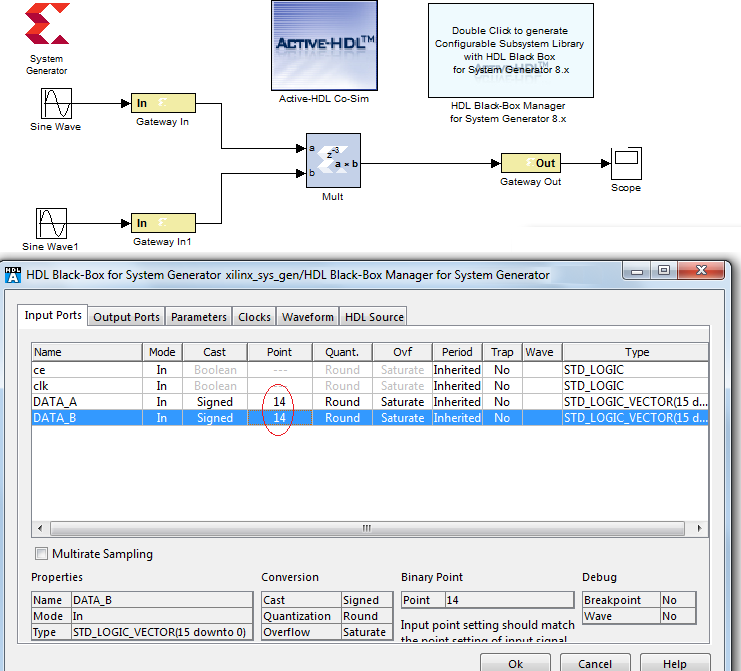8. Select the Clocks tab of the Active-HDL System Generation Black Box and click on Add to insert a source for both clock and clock_enable.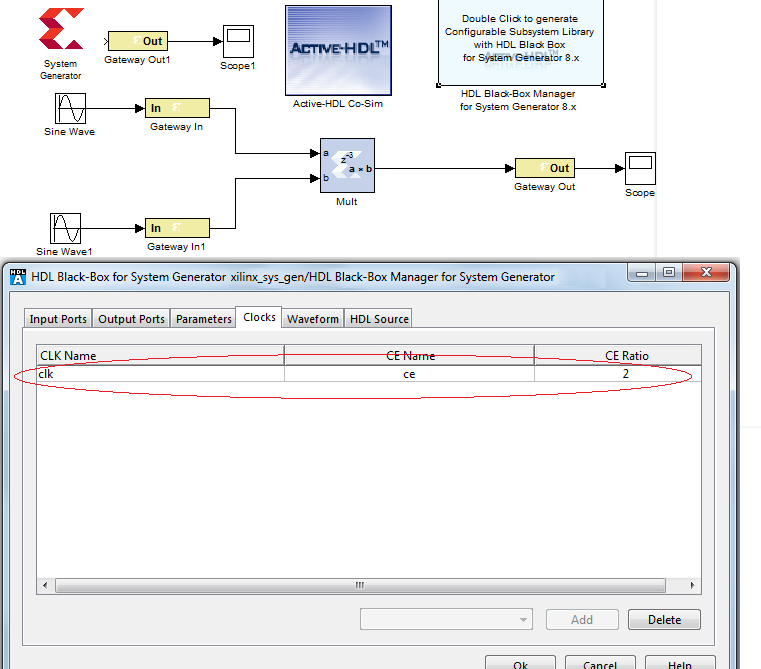9. Select the Waveform tab of the Active-HDL System Generation Black Box and click on Add to insert the selected waveform to be viewed in Active-HDL.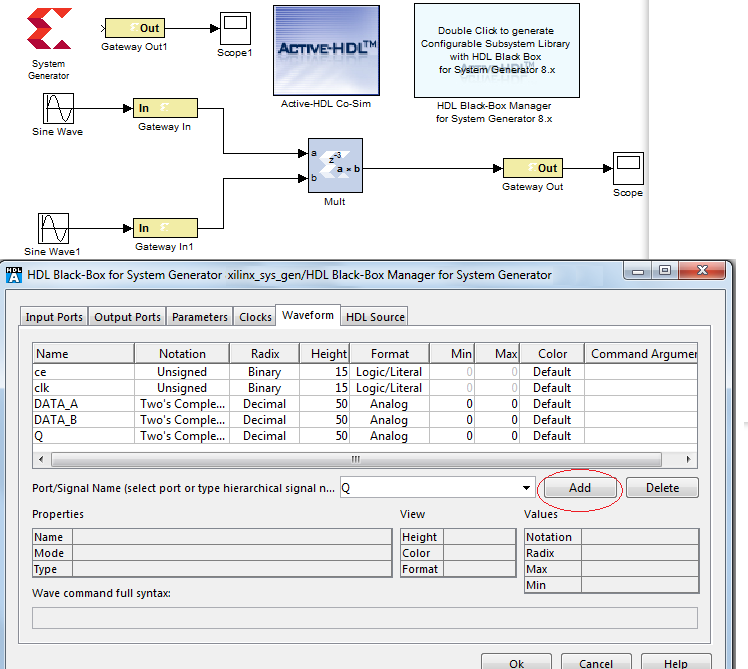10. Click OK to apply all changes to Active-HDL System Generation Black Box and 3 boxes will appear where only 1 will be used. Select the "_cfg" block and insert it into the model as shown with all connections in place. Remove the Active-HDL System Generation Black Box from the model.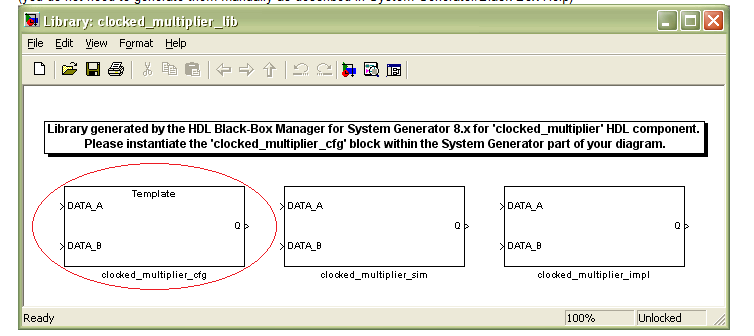11. Open the Xilinx System Generator by left-clicking on it and click the Generate tab so as to execute a build of all Xilinx parts used in the model.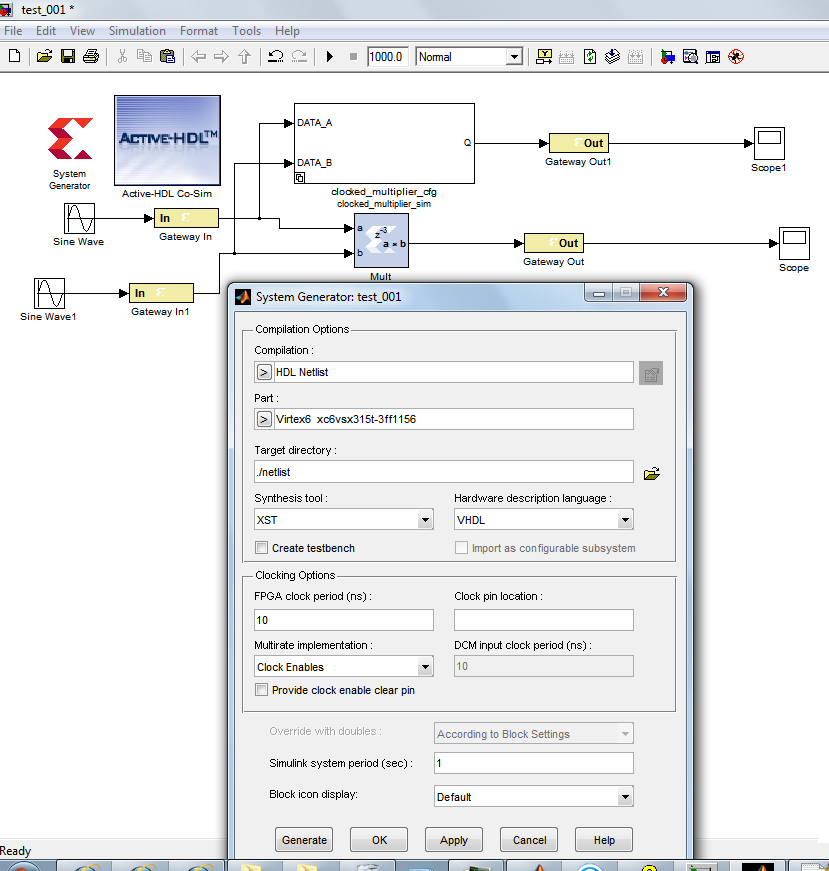12. Now run the MATLAB SIMULINK simulation and Active-HDL will automatically be launched after clicking the “CONTINUE” button. Step through the pre-load questions of Active-HDL and then a client connection will be made and the simulation is run until completion.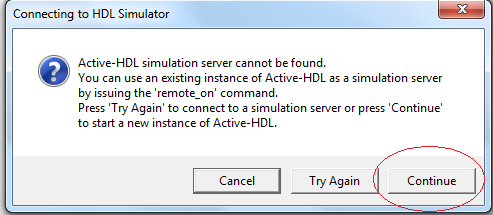The co-simulation will start and the model will run for 1000 msec or the desired run-time selected.

13. Observe the waveforms in Active-HDL.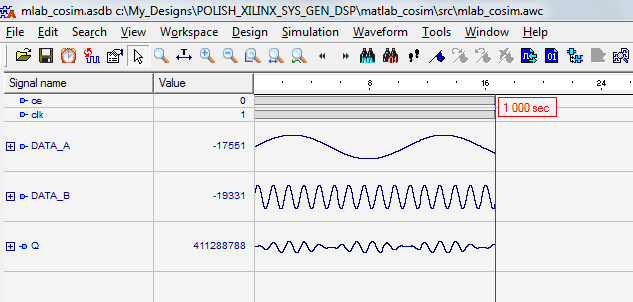14. Observe the scope results from executing the model. Compare the displays to ensure they are identical.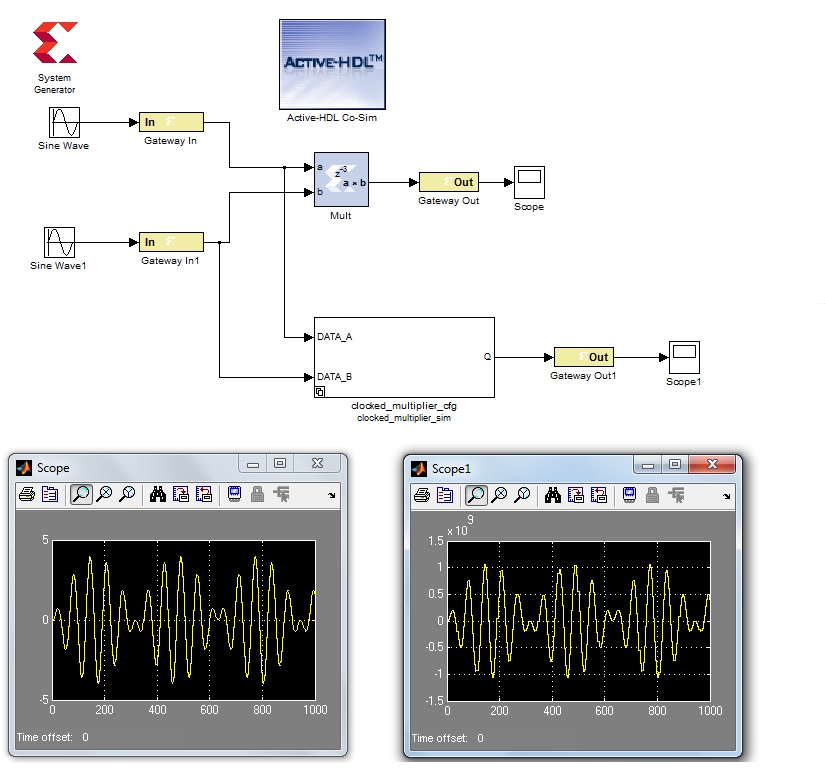1. Close the MATLAB SIMULINK model and return to Active-HDL and set the design active as shown in the figure.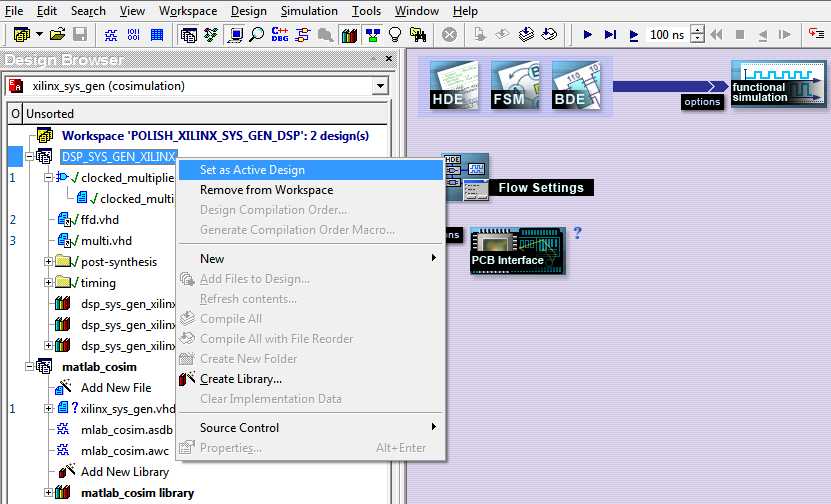2. Set the Xilinx Synthesis Options for the chosen FPGA and top level of design as shown in the figure.3. Synthesize the design and check the log reports for errors and warnings.4. Set the Xilinx Implementation Options and set the HDL to Verilog or VHDL for the Simulation Output Format as shown in the figure.5. Place and route the design without using Floor Planner and check the log reports for errors and warnings.## Preparing to do the Co-simulation with the Xilinx Timing file in Active-HDL

1. Open TIME_SIM.VHD and change the ROC_WIDTH at the end of the file to a value in milliseconds as shown.

```  NlwBlockROC : X_ROC
generic map (ROC_WIDTH => 100 ms)  --match this value
port map (O => GSR);
NlwBlockTOC : X_TOC
port map (O => GTS);
```
2. Compile the additional Active-HDL design files added from the Xilinx Synthesis & Implementation process as show in the figure. Set the Timing library active and select the entity/architecture pair as shown.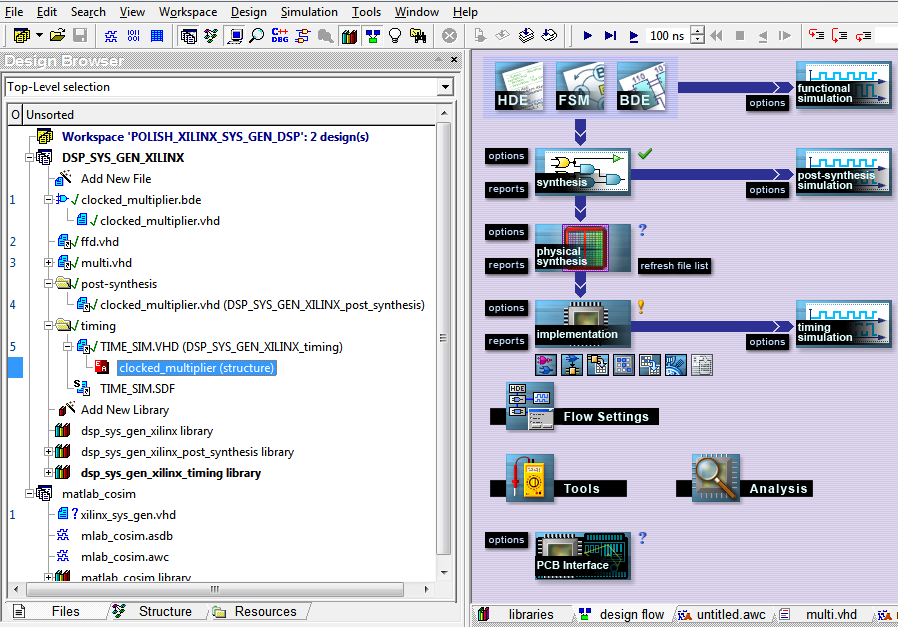3. Expand a source file (e.g. TIME_SIM.VHD) that contains the top level design unit that will be used as a HDL black-box in Simulink. Right-click on this unit (e.g. clocked_multiplier(structure)) and select the Generate Block Description for Simulink option from the pop-up menu.4. Press Save to complete the MATLAB M-File generation.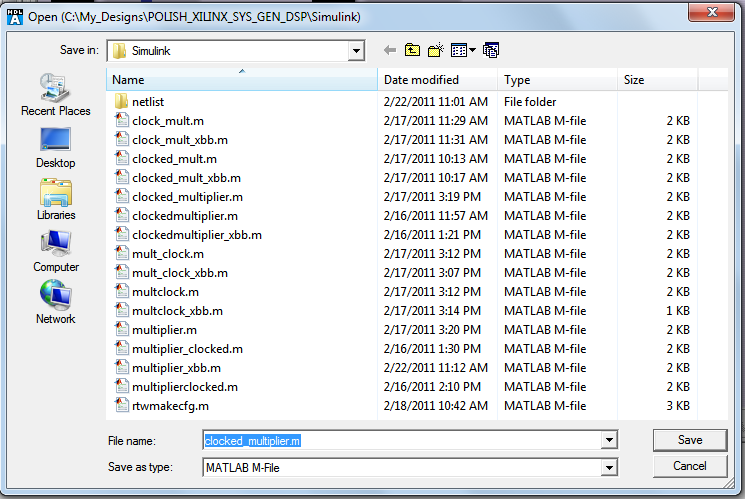You will see the following messages in the console:

# Generating block description file: 'C:\My_Designs\POLISH_XILINX_SYS_GEN_DSP\Simulink\clocked_multiplier.m'...

# Generation of block description file for "clocked_multiplier (structure)" completed successfully.

5. Repeat the steps for Pure System Generator Design using the new ".m" file generated from the Xilinx timing file.